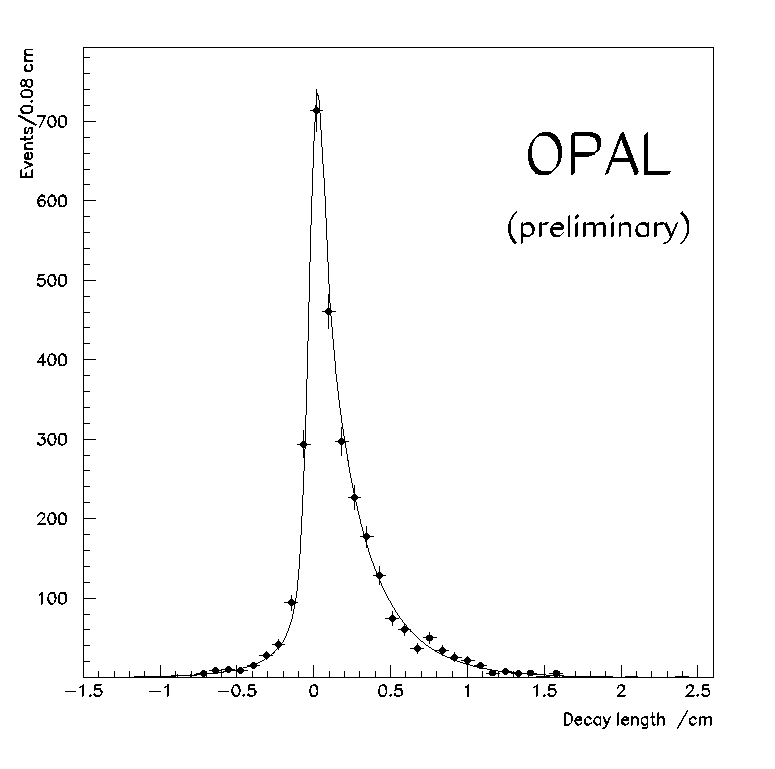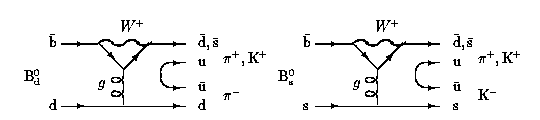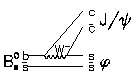# Exclusive B physics

OPAL has now recorded around 4 million hadronic decays of the Z0, of which some 22% involve the creation of a pair of heavy b-quarks. The B hadrons have many decay modes, but the statistics are now sufficient that we can identify significant numbers of events in channels where the B decay is completely (or almost completely) reconstructed. Several analyses at Cambridge are in this area.The OPAL microvertex detector is of great importance in identifying such decays, and allows us to measure the lifetimes of B hadrons. The relative lifetimes of B+ and B0 mesons are of theoretical interest, since this casts light on the underlying decay mechanism. The B mesons are tagged through decays into a charmed D meson and a lepton. A typical plot of the measured decay lengths of B mesons is shown above.

The tail of negative apparent lifetimes reflects the experimental resolution, while from the positive side we may infer the lifetimes:
1.62+-0.10+-0.10 for B0
1.53+-0.14+-0.11 for B+ .

Simple decays of B mesons to states like pi+pi-, K+K- or K+pi- are very rare, but very interesting. They may receive significant contributions from Penguin diagrams like these:A search for such decays in around 2 million Z decays has been performed, with negative results. A typical candidate is shown here. You can read the paper about this search by clicking here.

We are also investigating B mesons decays into the J/psi (charmonium) system. The J/psi decay can be identified very cleanly through its decay to electron or muon pairs. The J/psi can then be combined with other particles to reconstruct a B hadron decay. An example is the Bs meson containing a b quark and a strange antiquark. This can decay to J/psi + phi via a Feynman diagram like this:The phi meson decays to K+K-. A candidate event of this type may be seen if you click here. The two magenta tracks are probably K+ and K- produced from the decay of a phi meson, while the yellow tracks are muons from the decay of a J/psi. If we zoom in, we see that these tracks form a displaced vertex.